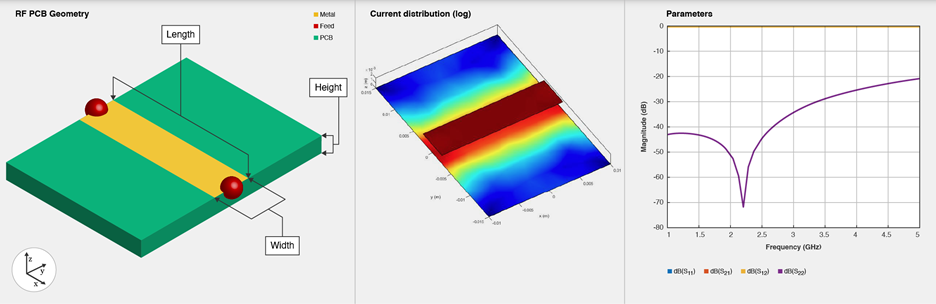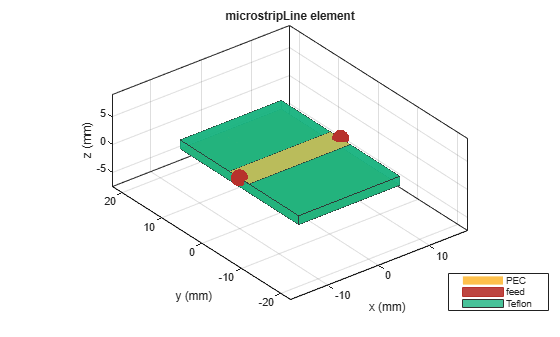# microstripLine

Create transmission line in microstrip form

## Description

Use the `microstripLine` object to create a microstrip transmission line. A microstrip line is a transmission line that is a basic building block for most RF planar microwave devices. You can use this transmission line to connect two PCB components or to create components such as filters, couplers, and feeding elements of various types of antennas.

Note

This PCB object supports behavioral modeling. For more information, see Behavioral Models. To analyze the behavioral model for a microstrip transmission line, set the `Behavioral` property in the `sparameters` function to `true or 1`

A few applications of microstrip transmission lines are:

• Creating matching feed and coupling networks

• Transmitting power from one component to another

• Feeding planar antennas and coupling structures

• Creating varying inductances or capacitances using open- or short ended- transmission lines## Creation

### Syntax

``microstrip = microstripLine``
``microstrip = microstripLine(Name=Value)``
``microstrip = microstripLine(txlineobj)``

### Description

example

````microstrip = microstripLine` creates a default microstrip transmission line using a Teflon substrate.```

example

````microstrip = microstripLine(Name=Value)` sets properties using one or more name value pair arguments. For example, ```microstrip = microstripLine(Length=0.0300)``` creates a microstrip line of length 0.0300 meters. Properties not specified retain their default values.```
````microstrip = microstripLine(txlineobj)` creates a microstrip transmission line from the behavioral model of a `txlineMicrostrip` object in RF Toolbox™.```

## Properties

expand all

Length of the microstrip line in meters, specified as a positive scalar.

Example: `microstrip = microstripLine(Length=0.0300)`

Data Types: `double`

Width of the microstrip line in meters, specified as a positive scalar.

Example: `microstrip = microstripLine(Width=0.00630)`

Data Types: `double`

Height of the microstrip line from the ground plane in meters, specified as a positive scalar.

In the case of a multilayer substrate, you can use the height property to create a microstrip line at the interface of the two dielectrics.

Example: `microstrip = microstripLine(Height=0.0015)`

Data Types: `double`

Width of the ground plane in meters, specified as a positive scalar.

Example: `microstrip = microstripLine(GroundPlaneWidth=0.0400)`

Data Types: `double`

Type of dielectric material used as a substrate, specified as a `dielectric` object. For more information, see `dielectric`. The thickness of the default dielectric material Teflon is `0.0016` m or the same as the height property.

Example: `d = dielectric('FR4');` `microstrip = microstripLine(Substrate=d)`

Data Types: `string` | `char`

Type of metal used in conducting layers, specified as a `metal` object. For more information see `metal`.

Example: `m = metal('PEC');` `microstrip = microstripLine(Conductor=m)`

Data Types: `string` | `char`

## Object Functions

 `charge` Calculate and plot charge distribution `current` Calculate and plot current distribution `design` Design microstrip transmission line around specified frequency `feedCurrent` Calculate current at feed port `getZ0` Calculate characteristic impedance of transmission line `layout` Plot all metal layers and board shape `mesh` Change and view mesh properties of metal or dielectric in PCB component `shapes` Extract all metal layer shapes of PCB component `show` Display PCB component structure or PCB shape `sparameters` Calculate S-parameters for RF PCB objects

## Examples

collapse all

Create and view a default microstrip transmission line.

`microstrip = microstripLine`
```microstrip = microstripLine with properties: Length: 0.0200 Width: 0.0050 Height: 0.0016 GroundPlaneWidth: 0.0300 Substrate: [1x1 dielectric] Conductor: [1x1 metal] ```
`show(microstrip)`Design a microstrip transmission line at 3 GHz, with a characteristic impedance of 70 ohms and a line length 1.5 times the wavelength.

`microstrip = design(microstripLine,3e9,'Z0',70,'LineLength',1.5)`
```microstrip = microstripLine with properties: Length: 0.1132 Width: 0.0030 Height: 0.0016 GroundPlaneWidth: 0.0150 Substrate: [1x1 dielectric] Conductor: [1x1 metal] ```

View the microstrip transmission line.

`show(microstrip)`Create and view a multilayer dielectric microstrip transmission line.

```microstrip = microstripLine; microstrip.Substrate = dielectric('Name',{'Teflon','Teflon'},'EpsilonR', ... [2.1 2.1],'LossTangent',[0 0],'Thickness',[0.8e-3 0.8e-3]); microstrip.Height = 0.8e-3; show(microstrip);```Design a microstrip transmission line at 3 GHz for FR4 substrate.

```d = dielectric('FR4'); d.LossTangent = 0; m = design(microstripLine('Substrate',d),3e9,'Z0',75,... 'LineLength',0.5);```

Create a microstrip cross.

```layer2d = traceCross('Length',[m.Length m.Length], ... 'Width',[m.Width m.Width]);```

Convert the cross trace to a PCB component.

```robj = pcbComponent(layer2d); robj.BoardThickness = m.Substrate.Thickness; robj.Layers{2} = m.Substrate; show(robj)```Define frequency points to calculate the s-parameters.

`freq = (1:3:40)*100e6;`

Calculate the s-parameters of the cross trace using the behavioral model.

`Sckt = sparameters(robj,freq,'Behavioral',true);`
```Warning: Behavioral model is valid only when EpsilonR is 9.9. ```

Calculate the s-parameters of the cross trace using the electromagnetic solver.

`Sem = sparameters(robj,freq);`

References:

1. Ramesh Garg & I. J. Bahl (1978) Microstrip discontinuities, International Journal of Electronics, 45:1, 81-87, DOI: 10.1080/00207217808900883

2. Wadell, Brian C. Transmission Line Design Handbook. The Artech House Microwave Library. Boston: Artech House, 1991.

Create mitered bend microstrip.

```m = design(microstripLine,6e9,"Z0",75); layer2d = bendMitered('Length',[m.Length/2 m.Length/2],... "Width",[m.Width m.Width],'MiterDiagonal',sqrt(2)*m.Width); robj = pcbComponent(layer2d); robj.BoardThickness = m.Substrate.Thickness; robj.Layers{2} = m.Substrate; show(robj)```Compute and plot s-parameters.

```freq = (1:2:60)*100e6; Sckt = sparameters(robj,freq,'Behavioral',true); Sem = sparameters(robj,freq); rfplot(Sckt,1,1,'db','-s') hold on rfplot(Sem,1,1,'db','-x')```Reference:

M. Kirschning, R. H. Jansen and N. H. L. Koster, "Measurement and Computer-Aided Modeling of Microstrip Discontinuities by an Improved Resonator Method," 1983 IEEE MTT-S International Microwave Symposium Digest, Boston, MA, USA, 1983, pp. 495-497, doi: 10.1109/MWSYM.1983.1130959.

 Pozar, David M. Microwave Engineering. 4th ed. Hoboken, NJ: Wiley, 2012.